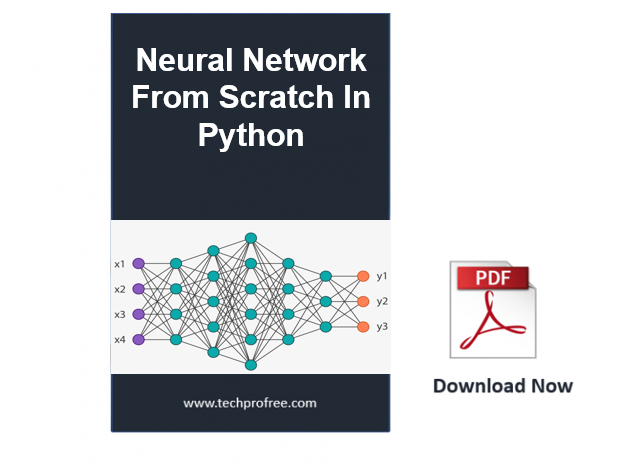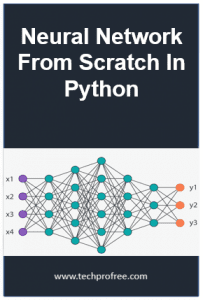Programming Books

# Neural Network From Scratch In PythonDownload free Introduction to Neural Networks for Beginners in PDF. This notes consists of Part A of a much larger, forth coming book “From o to Tensor Flow”. The aim of this much larger book is to get you up to speed with all you get to start on the deep learning journey.

From Starting To TensorFlow then Deep Learning. as we Know Deep learning is part of Machine learning Then the Basic Concept of Neural network and how we can create in python .

This notes covers getting you up to speed up on the basic concept of neural networks and how to create them in python.

What is Artificial Neural Networks

Simple Definition of Artificial Neural network is software implementations of the neuronal structure of our brains.

 Notes Neural Networks From Scratch in Python Type PDF Language English Language English Addition With Real-Time Examples

You Learn These Topics From This Notes:

1.Introduction

2. Introduction to Neural Networks

• The Structure of ANN
• The Artificial Neuron
• Nodes
• The Bias

3. The Feed-Forward Pass

• A Feed-Forward Example
• Our First Attempt at a Feed-Forward Function
• A More Efficient Implementation
• Vectorisation In Neural Network
• Matrix Multiplication

• A Simple Example in Code
• The Cost Function
• Gradient Descent in Neural Network
• A Two Dimensional Gradient Descent Example
• Propagating in to the Hidden Layers
• Back Propagation in Depth
• Implementation the Gradient Descent Step
• The Final Gradient Descent Algorithm

5. Implementing the Neural Network in Python

• Scaling Data
• Creating Test and Training Datasets
• Setting Up the Output Layers
• Creating the Neural Network
• Assessing the Accuracy of the Trained Model